Start typing, then use the up and down arrows to select an option from the list.Jules Bruno
130views
3
Hey guys in this new video, we're gonna continue with further calculations dealing with gibbs free energy. So in this example it says for mercury the entropy of vaporization equals 58.5 kg per mole. And then the entropy of vaporization equals 92.9 jewels over kelvin's times moles at 25 degrees Celsius. Now I'm asking you does mercury boil at 3300 and 50 degrees Celsius and one atmosphere pressure. Now this question is similar to something we've seen earlier. What I'm really asking you for is calculating the normal boiling point of mercury. Yeah, if we can find out the normal boiling point of mercury, we can see if this temperature is high enough for mercury to begin to boil. So remember if we're looking for the normal boiling point of mercury, we're gonna assume that delta G zero is equal to zero. And doing this, we're gonna say delta G zero equals delta H. Zero minus t delta S zero. Since this is equal to zero. We can now plug in all we know we can plug in the delta H value as well as the delta S value. So it's similar to something we've seen earlier. So this will be 58.5 kg jewels over moles minus t. We don't know temperature, we're looking for it times. Remember entropy of vaporization has to be in kila jules just like delta H. S and killed jules. So divide that by 1000 gives us 10000.9 to 9 kila jewels over k times moles. So we're gonna subtract 58.5 killer Joel's over moles. So negative 58.5 kg Joel's over moles equals negative temperature times 0.929 kila jewels over K. Times moles. We need to isolate our temperature. So we're gonna divide out negative zero 9 - nine. Kill jules over K. times moles. Okay, so when we do that, this cancel out with this, the negative sign cancels out. We're gonna say that killer jewels and moles will cancel with these killer jewels and moles leaving us with what units left kelvin. So temperature here equals 6 29.7 Kelvin. Okay, so we're gonna say here that is the normal boiling point of water. So that means the temperature has to be at minimum this temperature for mercury to begin to boil. So we go back to the 350°C. We have to change it to Kelvin. So add to 73.15 We get 6 23.15 Kelvin. And we can see that this temperature here is not high enough. It has to be a minimum of 6, 29.7. The temperature we calculated earlier. So we're gonna say no, it does not boil not hot enough has to be a little bit hotter than that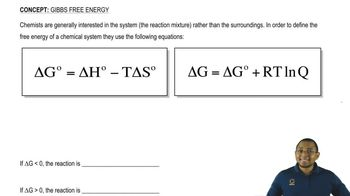06:13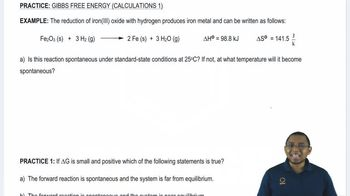06: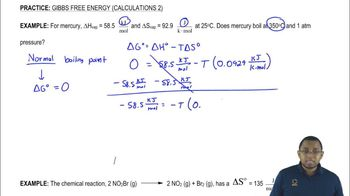03:26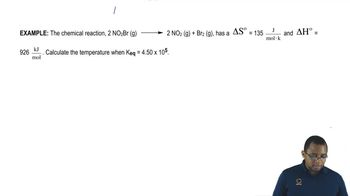04:08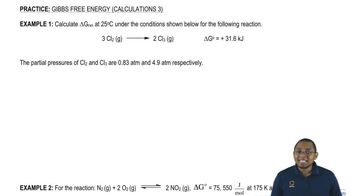02:37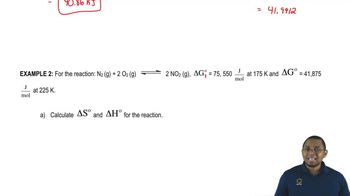04:01